A star is a graph traversal and path search algorithm, which is often used in computer science due to its completeness, optimality, and optimal efficiency.

A star is one of the most successful search algorithms to find the shortest path between nodes or graphs. It is an informed search algorithm, as it uses information about path cost and also uses heuristics to find the solution. You can read more about this at towardsdatascience.com.

## Let’s learn how A star search algorithm works

A star evaluates nodes by combining g(n), the cost to reach the node, and h(n), the cost to get from the node to the goal: f(n) = g(n) + h(n) .

Since g(n) gives the path cost from the start node to node n, and h(n) is the estimated cost of the cheapest path from n to the goal, we have f(n) = estimated cost of the cheapest solution through n.

Thus, if we are trying to find the cheapest solution, a reasonable thing to try first is the node with the lowest value of g(n) + h(n).

### Problem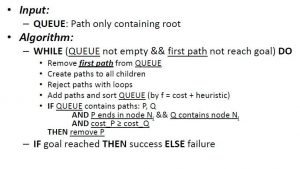Image 1: Pseudo Code of an A Star Algorithm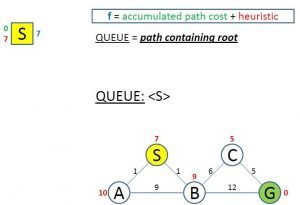Image 2: The Problem of A Star Search Algorithm

On Image 2 we have the problem that we are going to use to show you how A star search algorithm works. The problem consists of finding the shortest path from the start node “S” to the goal node “G”.

As we can see from the image, the total cost (in blue color on the right of the node) is calculated by adding the value of the cost to reach to node n (in red color on the left of the node) and the heuristic value (in green color on the left of the node) which the estimated cost of the cheapest path from n to the goal.

From the starting point, we have a total cost of 7 since we don’t have any cost to reach to node “S” from node “S” and the heuristic from “S” to “G” is 7.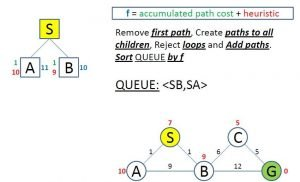Image 3: First Evaluation of A Star Algorithm

On Image 3 we can see the first evaluation. We can see that the first nodes connected to the start node are visited. Both of these nodes are connected to other nodes.

So, for now, we will continue evaluating the node with the lowest total cost. In this case that is the node “B”.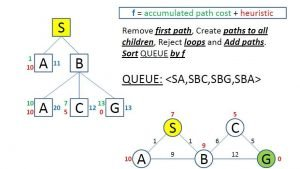Image 4: Second Evaluation Through B

From node “B” we’ve created paths to other nodes, “A”, “C” and “G” which means we’ve reached the goal for the first time. We can see that the total cost of these nodes evaluated through the node “B” is calculated by adding the cost to reach from “S” to “B” plus the cost to reach from “B” to each of the other nodes.

We can also see that now, all of these nodes have a higher total cost that the node “A” that we’ve visited in the first evaluation.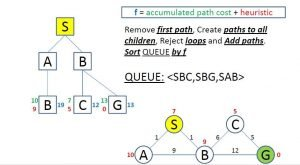Image 5: Third Evaluation Through A

On Image 5 is shown the third evaluation, where we are going through the node “A”. We can see that from node “A” we can reach only to node “B” and that now the total cost is 19, which is higher than the total cost of the other nodes.

We can also see that we removed the path SBA since the total cost was the highest and we will never evaluate through that path.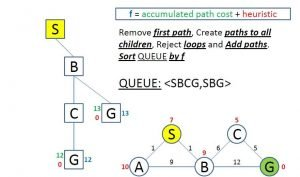Image 6: Evaluation Through C

On Image 6 we can see that we’ve also removed the path SAB since it had the highest total cost and that way it will never be used. In this evaluation through the node “C” we’ve reached the goal for the second time.

The total cost of reaching the goal is 12 (the heuristic here is 0 since you don’t need to estimate the cost of reaching the goal from the goal), which is lower than the total cost of the “G” node in the SBG path. Now we remove the SBG path, and we are left only with one path which is our solution.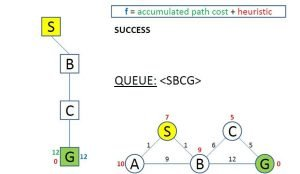Image 7: Successfully Reached The Goal Node

On Image 7 we can see that there is only one path in the queue, and that is the path with the lowest total cost of all, and that is our solution for this problem.

## Conclusion

A star is one of the most popular solutions for many cases where it can be applied, even though it can be outperformed by algorithms that can pre-process the graph to attain better performance.

Learning how this algorithm works, will help you understand how can you search in graphs in very efficient ways.

Like with every post we do, we encourage you to continue learning, trying and creating.

Don’t forget to read other articles about Data Science, Deep Learning and Computer Vision.